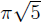# BBP-type formulas, in general bases, for arctangents of real numbers

Notes on Number Theory and Discrete Mathematics, ISSN 1310-5132
Volume 19, 2013, Number 3, Pages 33—54

## Details

### Authors and affiliations

Department of Physics, Obafemi Awolowo University
Ile-Ife, Nigeria

Olawanle Layeni
Department of Mathematics, Obafemi Awolowo University
Ile-Ife, Nigeria

### Abstract

BBP-type formulas are usually discovered experimentally, one at a time and in specific bases, through computer searches using PSLQ or other integer relations finding algorithms. In this paper, however, we give a systematic analytical derivation of numerous new explicit digit extraction BBP-type formulas for the arctangents of real numbers in general bases. Our method has the clear advantage that the proofs of the formulas are contained in the derivations, whereas in the experimental approach, proofs of discovered formulas have to be found separately. The high point of this paper is perhaps the discovery, for the first time, of a BBP-type formula for.

### Keywords

• BBP-type formulas
• Polyalgorithm constants
• Digit extraction formulas

• 11Y60
• 30B99

### References

1. Lord, N. Recent formulae for p: Arctan revisited! The Mathematical Gazette, Vol. 83, 1999, 479–483.
2. Bailey, D. H. A compendium of bbp-type formulas for mathematical constants, February 2011. Available Online http://crd.lbl.gov/~dhbailey/dhbpapers/bbp-formulas.pdf
3. Bailey, D. H., P. B. Borwein, S. Plouffe. On the rapid computation of various polylogarithmic constants. Mathematics of Computation, Vol. 66, 1997, No. 218, 903–913.
4. Bailey, D. H., R. E. Crandall. On the random character of fundamental constant expansions. Experimental Mathematics, Vol. 10, 2001, p. 175.
5. Borwein, J. M., D. Borwein, W. F. Galway. Finding and excluding b-ary machin-type BBP formulae, 2002. Available Online http://crd.lbl.gov/~dhbailey/dhbpapers/machin.pdf
6. Chamberland, M. Binary bbp-formulae for logarithms and generalized Gaussian–Mersenne primes. Journal of Integer Sequences, Vol. 6, 2003.
7. Adegoke, K., J. O. Lafont, O. Layeni. A class of digit extraction BBP-type formulas in general binary bases. Notes on Number Theory and Discrete Mathematics, Vol. 17, 2011, No. 4, 18–32.

## Cite this paper

Adegoke, K., & Layeni, O. (2013). BBP-type formulas, in general bases, for arctangents of real numbers. Notes on Number Theory and Discrete Mathematics, 19(3), 33-54.